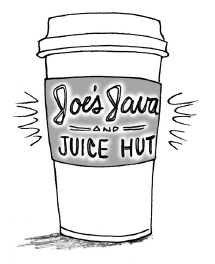### Home > CCG > Chapter 10 > Lesson 10.3.3 > Problem10-142

10-142.Of the $63$ drinks at Joe’s Java and Juice Hut, $42$ contain coffee and $21$ contain dairy products. If Alexei randomly chooses a drink, what is the probability of getting a drink with both coffee and a dairy product? What is the probability of getting neither coffee nor a dairy product? Assume that choosing a coffee drink is independent of choosing a dairy-product drink. See if you can answer these questions without making a two-way table first.

If events A and B are independent, the $\text{P}(\text{A and B})=\text{P}(\text{A})·P(\text{B})$.

If $\text{P}(\text{coffee})=\frac{42}{63}$, what is $\text{P}(\text{no coffee})$?

If $\text{P}(\text{dairy})=\frac{42}{63}$, what is $\text{P}(\text{no dairy})$?